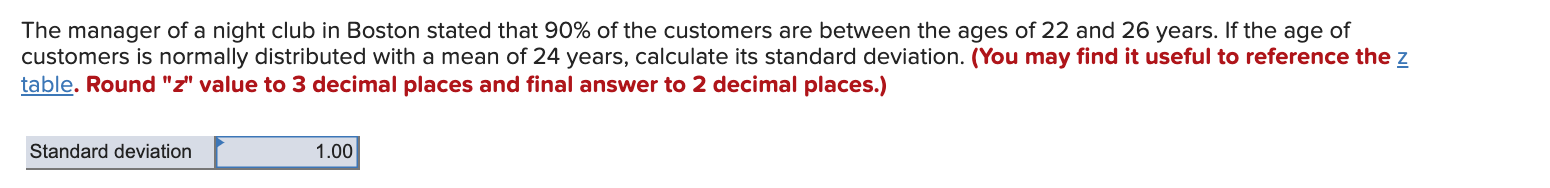# Question The manager of a night club in Boston stated that 90% of the customers are between the ages of 22 and 26 years. If the age of customers is normally distributed with a mean of 24 years, calculate its standard deviation. (You may find it useful to reference the z table. Round "z" value to 3 decimal places and final answer to 2 decimal places.) Standard deviation 1.00BEZZYH The Asker · Probability and StatisticsTranscribed Image Text: The manager of a night club in Boston stated that 90% of the customers are between the ages of 22 and 26 years. If the age of customers is normally distributed with a mean of 24 years, calculate its standard deviation. (You may find it useful to reference the z table. Round "z" value to 3 decimal places and final answer to 2 decimal places.) Standard deviation 1.00
More
Transcribed Image Text: The manager of a night club in Boston stated that 90% of the customers are between the ages of 22 and 26 years. If the age of customers is normally distributed with a mean of 24 years, calculate its standard deviation. (You may find it useful to reference the z table. Round "z" value to 3 decimal places and final answer to 2 decimal places.) Standard deviation 1.00